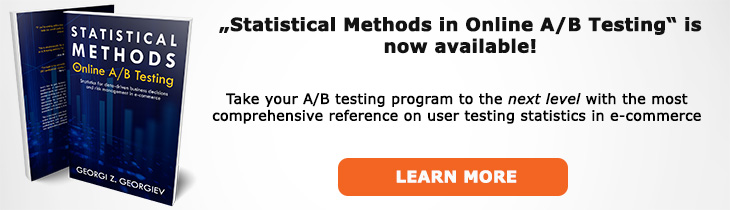# What does "p-value" mean?

Definition of p-value in the context of A/B testing (online controlled experiments).

## What is p-value?

The p-value, denoted by the small letter "p", is the probability of observing a test statistic as extreme or more extreme than the observed under the assumption that the null hypothesis is true. It is a post-hoc statistic meaning that it can only be computed after a test is completed (or at intervals with appropriate p-value adjustments). In proper notation it is p = P(d(X) ≥ d(x0); H0) where P stands for probability, d(X) is a test statistic (distance function) x0 is a typical realization of X and H0 is the selected null hypothesis. The distance function often comes in the form of a t Score or a z Score.

One can think of the p-value as a summary statistic that encompasses information about the relation between the size of the observed difference between two or more test groups, the sample size, and the characteristics of the frequency distribution and thus the variance of the parameter of interest.

The p-value is usually viewed as a measure of how surprising a result is under the assumption that the null hypothesis is true. When we define a significance threshold past which we consider a result so unexpected that we are willing to reject the null hypothesis we can compare the observed significance level (p-value) with the threshold and if the latter is lower we can reject the null.

The interpretation of the p-value uses a probabilistic variant of the modus tollens logic: H->e, not-e ∴ not-H. Another way to interpret it is as a strong argument from coincidence: there was a low probability that something would have happened assuming the null was true, it did happen so it has to be an unusual (to the extend that the p-value is low) coincidence that it happened, warranting the conclusion to reject the null hypothesis. In an A/B testing context observing a p-value below the significance threshold means that we would implement a variant in the place of the current state of affairs (we have a "winner").

In terms of the predesignated type I error rate alpha observing a given p-value means that we would have rejected the null for any level α which is greater than the observed p-value.

In terms of confidence intervals, observing a given p-value means that a confidence interval with a confidence level greater than (1 - p) would not not cover the null hypothesis, for example if the p-value is 0.01 a confidence interval at a level less than 99% level would not include values under the null.

## Articles on p-value

Like this glossary entry? For an in-depth and comprehensive reading on A/B testing stats, check out the book "Statistical Methods in Online A/B Testing" by the author of this glossary, Georgi Georgiev.## Glossary Index by Letter

Select a letter to see all A/B testing terms starting with that letter or visit the Glossary homepage to see all.